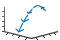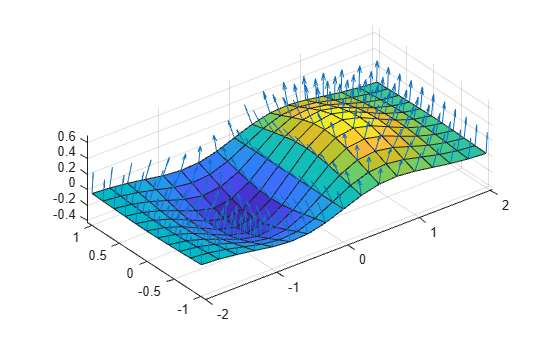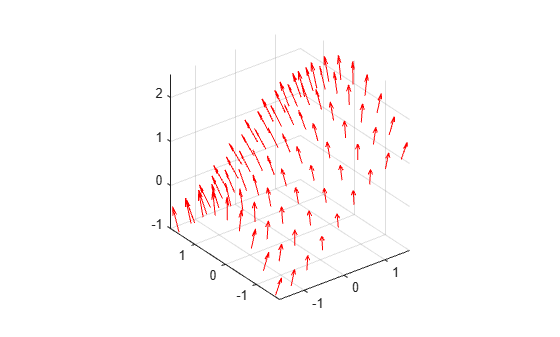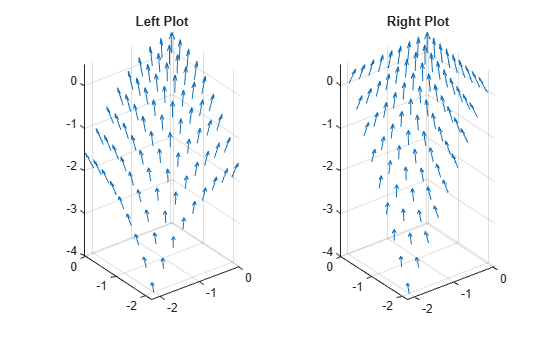# quiver3

3-D quiver or vector plot

•## Syntax

``quiver3(X,Y,Z,U,V,W)``
``quiver3(Z,U,V,W)``
``quiver3(___,scale)``
``quiver3(___,LineSpec)``
``quiver3(___,LineSpec,'filled')``
``quiver3(___,Name,Value)``
``quiver3(ax,___)``
``q = quiver3(___)``

## Description

example

````quiver3(X,Y,Z,U,V,W)` plots arrows with directional components `U`, `V`, and `W` at the Cartesian coordinates specified by `X`, `Y`, and `Z`. For example, the first arrow originates from the point `X(1)`, `Y(1)`, and `Z(1)`, extends in the direction of the x-axis according to `U(1)`, extends in the direction of the y-axis according to `V(1)`, and extends in the direction of the z-axis according to `W(1)`. By default, the `quiver3` function scales the arrow lengths so that they do not overlap.```
````quiver3(Z,U,V,W)` plots arrows with directional components specified by `U`, `V`, and `W` at equally spaced points along the surface `Z`. If `Z` is a vector, then the x-coordinates of the arrows range from 1 to the number of elements in `Z` and the y-coordinates are all 1.If `Z` is a matrix, then the x-coordinates of the arrows range from 1 to the number of columns in `Z` and the y-coordinates range from 1 to the number of rows in `Z`. ```

example

````quiver3(___,scale)` adjusts the length of arrows: When `scale` is a positive number, the `quiver3` function automatically adjusts the lengths of arrows so they do not overlap, then stretches them by a factor of `scale`. For example, a `scale` of 2 doubles the length of arrows, and a `scale` of 0.5 halves the length of arrows.When `scale` is `'off'` or `0`, such as `quiver3(X,Y,Z,U,V,W,'off')`, then automatic scaling is disabled. ```

example

````quiver3(___,LineSpec)` sets the line style, marker, and color. Markers appear at the points specified by `X`, `Y`, and `Z`. If you specify a marker using `LineSpec`, then `quiver3` does not display arrowheads. To specify a marker and display arrowheads, set the `Marker` property instead.```
````quiver3(___,LineSpec,'filled')` fills the markers specified by `LineSpec`.```
````quiver3(___,Name,Value)` specifies quiver properties using one or more name-value pair arguments. For a list of properties, see Quiver Properties. Specify name-value pair arguments after all other input arguments. Name-value pair arguments apply to all of the arrows in the quiver plot.```

example

````quiver3(ax,___)` creates the quiver plot in the axes specified by `ax` instead of the current axes (`gca`). The argument `ax` can precede any of the input argument combinations in the previous syntaxes.```

example

````q = quiver3(___)` returns a `Quiver` object. This object is useful for controlling the properties of the quiver plot after creating it.```

## Examples

collapse all

Load sample data that represents air currents over North America. For this example, select a subset of the data.

```load wind X = x(5:10,20:25,6:10); Y = y(5:10,20:25,6:10); Z = z(5:10,20:25,6:10); U = u(5:10,20:25,6:10); V = v(5:10,20:25,6:10); W = w(5:10,20:25,6:10);```

Create a 3-D quiver plot of the subset you selected. The vectors `X`, `Y`, and `Z` represent the location of the base of each arrow, and `U`, `V`, and `W` represent the directional components of each arrow. By default, the `quiver3` function shortens the arrows so they do not overlap. Call `axis equal` to use equal data unit lengths along each axis. This makes the arrows point in the correct direction.

```quiver3(X,Y,Z,U,V,W) axis equal```By default, the `quiver3` function shortens arrows so they do not overlap. To disable automatic scaling so that arrow lengths are determined entirely by `U`, `V`, and `W`, set the `scale` argument to `0`.

For example, first return the x-, y-, and z-coordinates of a unit sphere with 10-by-10 faces. Calculate the directional components of its surface normals using the `surfnorm` function. Then, create a 3-D quiver plot with no automatic scaling.

```[X,Y,Z] = sphere(10); [U,V,W] = surfnorm(X,Y,Z); quiver3(X,Y,Z,U,V,W,0) axis equal```For comparison, create the plot with automatic scaling. Note that the arrows are shorter and do not overlap.

```figure quiver3(X,Y,Z,U,V,W) axis equal```Plot vectors that are normal to the surface defined by the function $z=x{e}^{-{x}^{2}-{y}^{2}}$. Use the `quiver3` function to plot the vectors and the `surf` function to plot the surface.

First, create a grid of x- and y-values that are equally spaced. Use them to calculate z. Then, find the normal vectors.

```[X,Y] = meshgrid(-2:0.25:2,-1:0.2:1); Z = X.*exp(-X.^2 - Y.^2); [U,V,W] = surfnorm(X,Y,Z);```

Display the vectors as a 3-D quiver plot. Then, display the surface in the same axes. Adjust the display so that the vectors appear normal to the surface by calling `axis equal`.

```quiver3(X,Y,Z,U,V,W) hold on surf(X,Y,Z) axis equal```Create a 3-D quiver plot and specify a color for the arrows.

For example, first return the x-, y-, and z- coordinates of a surface. Calculate the directional components of its surface normals using the `surfnorm` function.

```[X,Y] = meshgrid(-pi/2:pi/8:pi/2,-pi/2:pi/8:pi/2); Z = sin(X) + cos(Y); [U,V,W] = surfnorm(Z);```

Then, create a 3-D quiver plot with red arrows.

```quiver3(X,Y,Z,U,V,W,'r') axis equal```Starting in R2019b, you can display a tiling of plots using the `tiledlayout` and `nexttile` functions. Call the `tiledlayout` function to create a 1-by-2 tiled chart layout. Call the `nexttile` function to create an axes object and return the object as `ax1`. Create the left plot by passing `ax1` to the `quiver3` function. Add a title to the plot by passing the axes to the `title` function. Repeat the process to create the right plot.

```[X,Y] = meshgrid(-2:0.25:0,-2:0.25:0); Z1 = -0.5*(X.^2 + Y.^2); [U1,V1,W1] = surfnorm(Z1); Z2 = -X.*Y; [U2,V2,W2] = surfnorm(Z2); tiledlayout(1,2) % Left plot ax1 = nexttile; quiver3(ax1,X,Y,Z1,U1,V1,W1) axis equal title(ax1,'Left Plot') % Right plot ax2 = nexttile; quiver3(ax2,X,Y,Z2,U2,V2,W2) axis equal title(ax2,'Right Plot')```Create a 3-D quiver plot and return the quiver object. Then, remove the arrowheads and add dot markers at the base of each arrow.

```[X,Y] = meshgrid(-3:0.5:3,-3:0.5:3); Z = 0.2*(Y.^2 - X.^2); [U,V,W] = surfnorm(Z); q = quiver3(X,Y,Z,U,V,W); axis equal q.ShowArrowHead = 'off'; q.Marker = '.';```## Input Arguments

collapse all

x-coordinates of the bases of arrows, specified as a scalar, a vector, or a matrix.

If `X` and `Y` are vectors and `Z`, `U`, `V`, and `W` are matrices, then `quiver3` expands `X` and `Y` into matrices. In this case, `size(Z)`, `size(U)`, `size(V)`, and `size(W)` must equal `[length(Y) length(X)]`. For more information about expanding vectors into matrices, see `meshgrid`.

If `X` and `Y` are matrices, then `X`, `Y`, `Z`, `U`, `V`, and `W` must be the same size.

y-coordinates of the bases of arrows, specified as a scalar, a vector, or a matrix.

If `X` and `Y` are vectors and `Z`, `U`, `V`, and `W` are matrices, then `quiver3` expands `X` and `Y` into matrices. In this case, `size(Z)`, `size(U)`, `size(V)`, and `size(W)` must equal `[length(Y) length(X)]`. For more information about expanding vectors into matrices, see `meshgrid`.

If `X` and `Y` are matrices, then `X`, `Y`, `Z`, `U`, `V`, and `W` must be the same size.

z-coordinates of the bases of arrows, specified as a scalar, a vector, or a matrix.

If `X` and `Y` are vectors and `Z` is a matrix, then `size(Z)` must equal `[length(Y) length(X)]`.

If `X` and `Y` are matrices, then `X`, `Y`, `Z`, `U`, `V`, and `W` must be the same size.

x-components of arrows, specified as a scalar, vector, or matrix.

If `X` and `Y` are vectors and `U` is a matrix, then `size(U)` must equal `[length(Y) length(X)]`.

If `X` and `Y` are matrices, then `X`, `Y`, `Z`, `U`, `V`, and `W` must be the same size.

y-components of arrows, specified as a scalar, vector, or matrix.

If `X` and `Y` are vectors and `V` is a matrix, then `size(V)` must equal `[length(Y) length(X)]`.

If `X` and `Y` are matrices, then `X`, `Y`, `Z`, `U`, `V`, and `W` must be the same size.

z-components of arrows, specified as a scalar, vector, or matrix.

If `X` and `Y` are vectors and `W` is a matrix, then `size(W)` must equal `[length(Y) length(X)]`.

If `X` and `Y` are matrices, then `X`, `Y`, `Z`, `U`, `V`, and `W` must be the same size.

Line style, marker, and color, specified as a character vector or string containing symbols. The symbols can appear in any order. You do not need to specify all three characteristics (line style, marker, and color).

If you specify a marker using `LineSpec`, then `quiver3` does not display arrowheads. To specify a marker and display arrowheads, set the `Marker` property instead.

Example: `'--or'` is a red dashed line with circle markers

Line StyleDescriptionResulting Line
`'-'`Solid line`'--'`Dashed line`':'`Dotted line`'-.'`Dash-dotted lineMarkerDescriptionResulting Marker
`'o'`Circle`'+'`Plus sign`'*'`Asterisk`'.'`Point`'x'`Cross`'_'`Horizontal line`'|'`Vertical line`'s'`Square`'d'`Diamond`'^'`Upward-pointing triangle`'v'`Downward-pointing triangle`'>'`Right-pointing triangle`'<'`Left-pointing triangle`'p'`Pentagram`'h'`HexagramColor NameShort NameRGB TripletAppearance
`'red'``'r'``[1 0 0]``'green'``'g'``[0 1 0]``'blue'``'b'``[0 0 1]``'cyan'` `'c'``[0 1 1]``'magenta'``'m'``[1 0 1]``'yellow'``'y'``[1 1 0]``'black'``'k'``[0 0 0]``'white'``'w'``[1 1 1]`Arrow scaling factor, specified as a positive number or `'off'`. By default, the `quiver3` function automatically scales the arrows so they do not overlap. The `quiver3` function applies the scaling factor after it automatically scales the arrows.

Specifying `scale` is the same as setting the `AutoScaleFactor` property of the quiver object. For example, specifying `scale` as `2` doubles the length of the arrows. Specifying `scale` as `0.5` halves the length of the arrows.

To disable automatic scaling, specify `scale` as `'off'` or `0`. When you specify either of these values, the `AutoScale` property of the quiver object is set to `'off'` and the length of the arrow is determined entirely by `U`, `V`, and `W`.

Target axes, specified as an `Axes` object. If you do not specify the axes, then the `quiver3` function uses the current axes.

### Name-Value Arguments

Specify optional comma-separated pairs of `Name,Value` arguments. `Name` is the argument name and `Value` is the corresponding value. `Name` must appear inside quotes. You can specify several name and value pair arguments in any order as `Name1,Value1,...,NameN,ValueN`.

Example: `'Color','r','LineWidth','1`

Note

The properties listed here are only a subset. For a complete list, see Quiver Properties.

Width of arrow stem and head, specified as a scalar numeric value greater than zero in point units. One point equals `1/72` inch. The default value is `0.5` point.

Example: `0.75`

Arrowhead display, specified as `'on'` or `'off'`, or as numeric or logical `1` (`true`) or `0` (`false`). A value of `'on'` is equivalent to `true`, and `'off'` is equivalent to `false`. Thus, you can use the value of this property as a logical value. The value is stored as an on/off logical value of type `matlab.lang.OnOffSwitchState`.

• `'on'` — Display the vectors with arrowheads.

• `'off'` — Display the vectors without arrowheads.

Automatic scaling of arrow length, specified as `'on'` or `'off'`, or as numeric or logical `1` (`true`) or `0` (`false`). A value of `'on'` is equivalent to `true`, and `'off'` is equivalent to `false`. Thus, you can use the value of this property as a logical value. The value is stored as an on/off logical value of type `matlab.lang.OnOffSwitchState`.

• `'on'` — Scale the arrow length to fit within the grid-defined coordinate data and scale arrows so that they do not overlap. The `quiver` or `quiver3` function then applies the `AutoScaleFactor` to the arrow length.

• `'off'` — Do not scale the arrow lengths.

Scale factor, specified as a scalar. A value of `2` doubles the length of the arrows. A value of `0.5` halves the length of the arrows.

This property has an effect only if the `AutoScale` property is set to `'on'`.

Example: `2`

## Tips

To create a 3-D quiver plot using cylindrical or spherical coordinates, first convert them to Cartesian coordinates using the `pol2cart` or `sph2cart` function.

## Extended Capabilities

### Topics

Introduced before R2006a